# BMI calculator

Create a BMI calculator in Excel and find out if you are a healthy weight. Body mass index (BMI) is a measure of body fat based on weight and height that applies to adult men and women. If you're in a hurry, simply download the Excel file.

Below you can find a screenshot of the BMI calculator.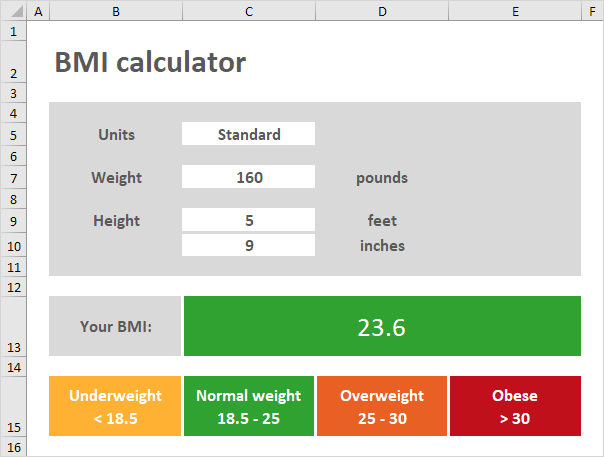Note: the instructions below do not teach you how to format the worksheet. We assume that you know how to change font sizes, font styles, insert rows and columns, add borders, change background colors, etc.

To create this BMI calculator, execute the following steps.

1. Create a drop-down list in cell C5.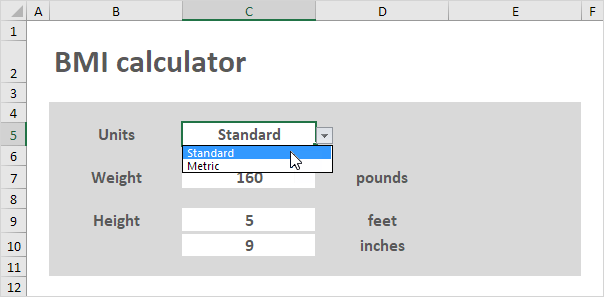2. Insert an IF function into cell D7, D9 and D10 to show the correct units.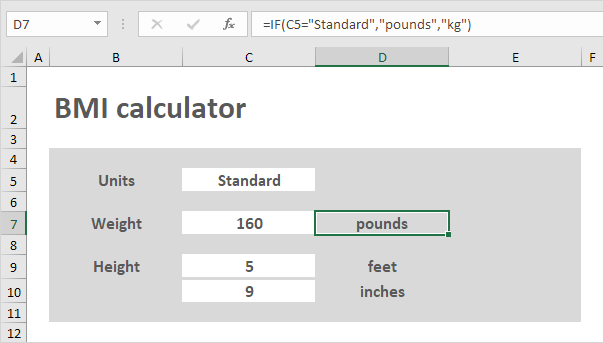3. Select Metric from the drop-down list. The IF function in cell D10 returns an empty string. Create a conditional formatting rule that changes the format of cell C10 when you select Metric from the drop-down list (step 6, 7 and 8).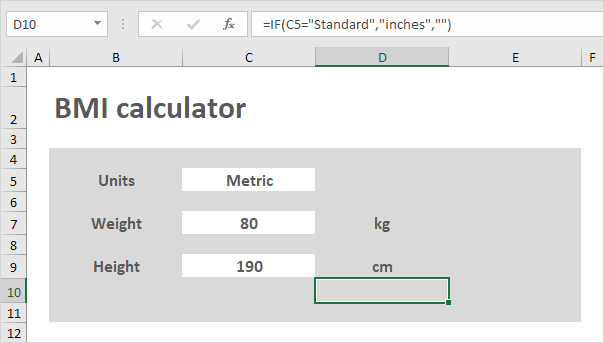4. Create the BMI formula.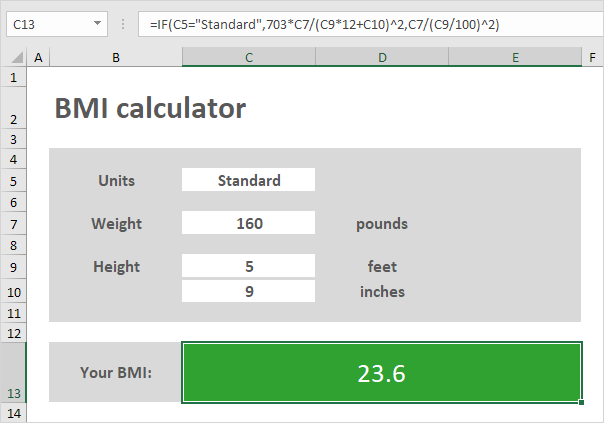Explanation: C9*12+C10 calculates the height in inches and C9/100 calculates the height in meters. Using standard units, BMI = 703*pounds/inches^2. Using metric units, BMI = kg/m^2. Excel uses a default order in which calculations occur. If a part of the formula is in parentheses, that part will be calculated first. Also remember, Excel performs exponentiation (^) before multiplication (*) and division (/).

5. Enter a weight of 250 pounds. Excel calculates the body mass index (BMI) and automatically changes the background color of the range C13:E13. This feature is called conditional formatting (step 6, 7 and 8).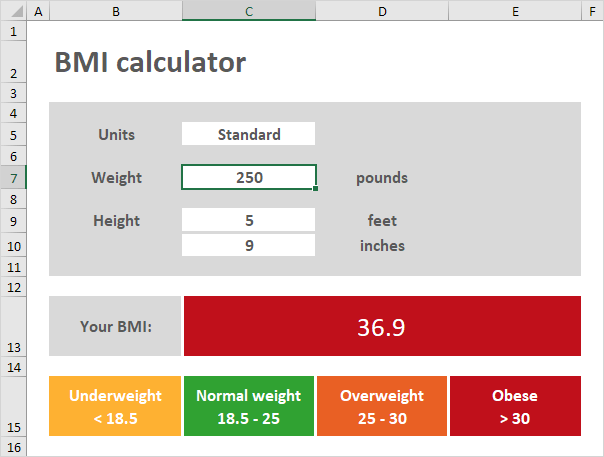6. On the Home tab, in the Styles group, click Conditional Formatting.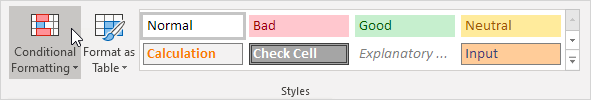7. Click Manage Rules.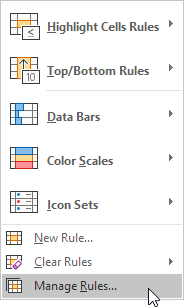The Conditional Formatting Rules Manager appears.

8. From the drop-down list, change Current Selection to This Worksheet, to view all conditional formatting rules in this worksheet.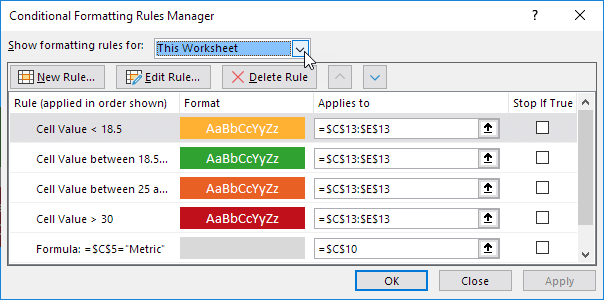Note: select a rule and click Edit Rule to take a closer look at each rule. The first 4 rules correspond with the BMI categories (underweight, normal weight, overweight and obese). The 5th rule changes the format of cell C10 when you select Metric from the drop-down list (step 3).

Go to Next Chapter: Data Validation